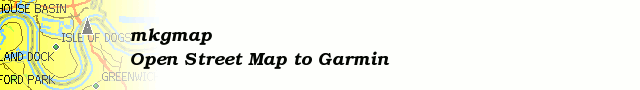# [mkgmap-dev] DEM format Questions

From Frank Stinner frank.stinner at leipzig.de on Fri Nov 10 10:48:53 GMT 2017

```Hi Gerd,

which 0-Bit you mean? Let us take this example:

4,4,4,5,7,7,7,7,7,7,7,7,7,7,7,7,7,7,7,7,7,7,7,7,7,7,7,7,7,7,7,7,7,7,7,7,7,7,7,7,7,7,7,7,7,7,7,7,7,7,7,7,7,7,7,7,7,7,7,7,7,7,7,7
8,8,5,5,5,5,5,8

The detailview from my prog "Input2" (
https://github.com/FSofTlpz/Garmin-DEM-Build/blob/master/Input2/bin/Input2.exe
) show us:

MaxHeigth=158, Codingtype=0, TileSize=64x64, BaseHeigthUnit=1,
HeigthUnit=0, ActualMode=notdefined, ActualHeigth=0
Line 0
Idx=0, Plateau Length=0 TableIdx=0 Bits=0 [.] <>
Idx=0, PlateauFollower ActualHeigth=4, Value=4 PlateauFollower0 [...11]
Hybrid1 ddiff=0
Idx=1, ActualHeigth=4, Value=0 Standard [1.] Hybrid1
...
Idx=62, ActualHeigth=7, Value=0 Standard  Length0
Idx=63, ActualHeigth=7, Value=0 Standard  Length0
Line 1
Idx=0, Plateau Length=0 TableIdx=0 Bits=0 [.] <>
Idx=0, PlateauFollower ActualHeigth=8, Value=4 PlateauFollower0 [.111]
Hybrid2 ddiff=0
Idx=1, ActualHeigth=8, Value=0 Standard  Length0
Idx=2, ActualHeigth=5, Value=-3 Standard [......1] Length0
Idx=3, Plateau Length=4 TableIdx=3 Bits=1 [1111..] <111a>
Idx=7, PlateauFollower ActualHeigth=8, Value=-1 PlateauFollower1 [.1.]
Hybrid1 ddiff=2

Between the brackets [ and ] are the bits (. means 0).

There is no special case. If a plateau have to begin, we have as first the
plateau-length (see "Codierung der Plateaulänge"). There is ever a 0-Bit
between the 2 parts of length-coding, also when plateau-length=0 (often on
start of a line).
As next we have the height-coding for the follower.

Frank
-------------- next part --------------
An HTML attachment was scrubbed...
URL: <http://www.mkgmap.org.uk/pipermail/mkgmap-dev/attachments/20171110/21304d17/attachment.html>
```